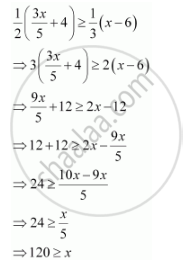CBSE (Arts) Class 11CBSE
Share

# Solve the Given Inequality for Real X: 1/2 (3x/5 + 4) >= 1/3 (X -6) - CBSE (Arts) Class 11 - Mathematics

ConceptAlgebraic Solutions of Linear Inequalities in One Variable and Their Graphical Representation

#### Question

Solve the given inequality for real x: 1/2 (3x/5 + 4) >= 1/3 (x -6)

#### SolutionThus, all real numbers x,which are less than or equal to 120, are the solutions of the given inequality.

Hence, the solution set of the given inequality is (–∞, 120].

Is there an error in this question or solution?

#### APPEARS IN

NCERT Solution for Mathematics Textbook for Class 11 (2018 to Current)
Chapter 6: Linear Inequalities
Q: 12 | Page no. 122
Solution Solve the Given Inequality for Real X: 1/2 (3x/5 + 4) >= 1/3 (X -6) Concept: Algebraic Solutions of Linear Inequalities in One Variable and Their Graphical Representation.
S